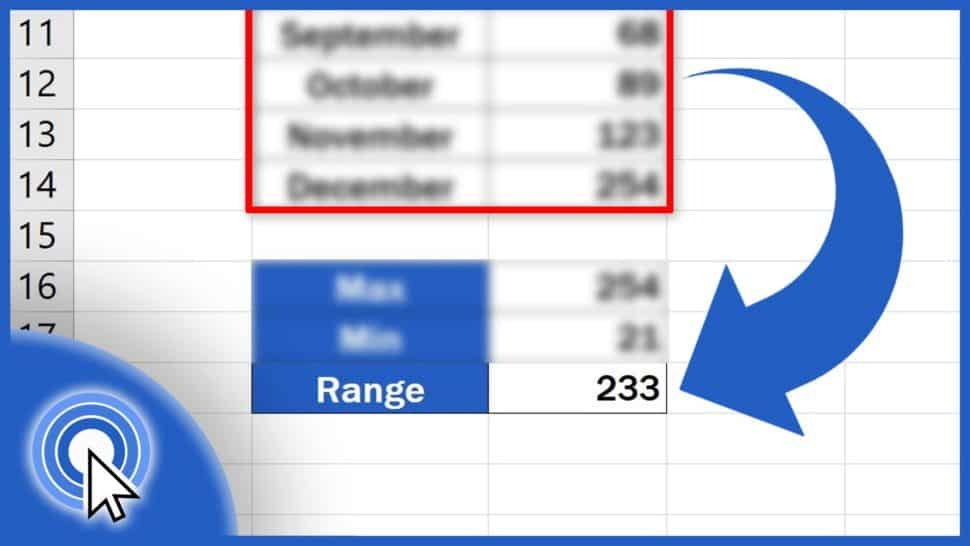# How to Calculate the Range in Excel (in 3 easy steps)In this tutorial, you’ll see three easy steps to calculate the range of a set of data in Excel, no matter how many values there are in the data table you’re working with.

Would you rather watch this tutorial? Click the play button below!

Contents

The range is the difference between the lowest and the highest value within a set of data. So, to calculate the range, first you’ll need to find out the maximum and the minimum value.

## The First Step – How to Find Out The Maximum Value

Let’s start with the first step, which is to find out the maximum value from this table of sales. Click into the selected cell, enter the equal sign and type in ‘MAX’. Excel will suggest a function. Select it and carry on defining what cells exactly should be taken into consideration when extracting this information.

Click on the cell C3, hold the left mouse button and drag the selection area down to C14.

Close the brackets, hit ‘Enter’ and that’s it for the first step. We can see that the maximum value from the table is displayed in the selected cell.

## The Second Step – How to Find Out The Minimum Value

The second step will be somewhat similar to the first one, but we’re going to look for the minimum value, so we’ll use the function ‘MIN’. Click on the cell, type in the equal sign, then ‘MIN’, select the function and mark the area with the relevant data as previously.

Close the brackets, press ‘Enter’, and the minimum value appears right where we need it!

## The Third Step – Using Formula That Will Easily Calculate The Range

Now, here comes the third and the last step of this tutorial. As we’ve already mentioned, the range can be calculated by subtracting the minimum value from the maximum value. We’ve got both now, so it’s gonna be quite easy.

Select the cell where you want the final number to appear, type in the equal sign again, and let’s enter the formula that will easily calculate the range. Enter the maximum value by clicking on the cell C16, type in the minus sign, which will subtract the minimum value stored in the cell C17, so click on C17 now.

Press ‘Enter’ and that’s all that’s needed! We’ve calculated the range in three easy steps!

If you’re thinking about the ways to present the data in a nice visual form, we’ve made some tutorials specifically on this topic. You can see them in the list below!

Don’t miss out a great opportunity to learn:

If you found this tutorial helpful, give us a like and watch other video tutorials by EasyClick Academy. Learn how to use Excel in a quick and easy way!

Is this your first time on EasyClick? We’ll be more than happy to welcome you in our online community. Hit that Subscribe button and join the EasyClickers!

Thanks for watching and I’ll see you in the next tutorial!